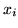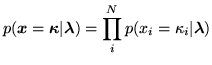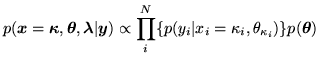Next: Non-spatial with Class Proportions Up: Discrete Labels Mixture Model Previous: Discrete Labels Mixture Model

### Non-spatial without Class Proportions

We assume spatial independence between the classification labels,, at each voxel:(2)

and that the prior on the discrete classification labels,, is a non-informative distribution with each class having equal probability. Using this in equation 1, the posterior becomes:(3)